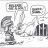T

##### Member
2019 Donor
VIP
I'm looking for a little help to fix this script I'm trying to create. I know it will be very simple for you guys as I'm still learning.

What I'm trying to do here is have the script draw 3 horizontal price lines (High, Low, Close) based on the manually inputted levels with a cloud around each level. Depending on the symbol displayed (either SPX or /ES) and depending on the trading session (RTH or ETH), I would also like to have the appropriate info as labels. So for example, if I have a chart of the SPX during Regular Trading Hours then it will have the lines and labels levels for SPX for the regular trading hour session. If I have a chart of the S&P 500 futures during RTH, then it will who the levels for /ES for RTH. If it's during the ETH session, then levels and label for the ETH session are shown instead. I will input the SPX levels for each session and the script will use that to come up with the /ES level based on the offset.

Here is the script. Let me know what I am doing wrong and if you have a better solution, please let me know.

Code:
``````#
# Algo Predicted High Low Close for RTH and ETH sessions #
# These levels are inputed manually and is only valid
# for the specified period!
#
# Offset is the difference between the SPX and S&P 500 Emini Futures in points

def na = Double.NaN;
def timeStrRTH = (SecondsFromTime(930) > 0) and (SecondsFromTime(1700) < 0);
def timeStrETH = ((1700) > 0) and (SecondsFromTime(930) < 0);

input AlgoHigh = 2801;
input AlgoLow = 2689;
input AlgoClose = 2743;
input AlgoHighETH = 2801;
input AlgoLowETH = 2689;
input AlgoCloseETH = 2743;
input offset = 12;
input MarginErrorHigh = 10;
input MarginErrorLow = 14.5;
input MarginErrorClose = 5;

def AHRTH = AlgoHigh - offset;
def ALRTH = AlgoLow - offset;
def ACRTH = AlgoClose - offset;
def AHETH = AlgoHighETH - offset;
def ALETH = AlgoLowETH - offset;
def ACETH = AlgoCloseETH - offset;

plot AH;
plot AL;
plot AC;

if  GetSymbol() == "/ES:XCME" {
AH = if timeStrRTH then AHRTH else if timeStrETH then AHETH else na ;
AL = if timeStrRTH then ALRTH else if timeStrETH then ALETH else na ;
AC = if timeStrRTH then ACRTH else if timeStrETH then ACETH else na ;
} else

if  GetSymbol() == "SPX" {
AH = if timeStrRTH then AlgoHigh else na ;
AL = if timeStrRTH then AlgoLow else na ;
AC = if timeStrRTH then AlgoClose else na ;
} else  {
AH = na;
AL = na;
AC = na;

}

def upperAH = AH + (MarginErrorHigh/2);
def lowerAH = AH - (MarginErrorHigh/2);
def upperAL = AL + (MarginErrorLow/2);
def lowerAL = AL - (MarginErrorLow/2);
def upperAC = AC + (MarginErrorClose/2);
def lowerAC = AC - (MarginErrorClose/2);

AH.SetDefaultColor(Color.RED);
AH.SetLineWeight(2);

AL.SetDefaultColor(Color.GREEN);
AL.SetLineWeight(2);

AC.SetDefaultColor(Color.ORANGE);
AC.SetLineWeight(2);

AddLabel(GetSymbolPart()== "SPX", "Algo High: " + AH, Color.RED);
AddLabel(GetSymbol()== "SPX" and timeStrRTH, "Algo Low: " + AlgoLow, Color.GREEN);
AddLabel(GetSymbol()== "SPX" and timeStrRTH, "Algo Close: " + AlgoClose, Color.ORANGE);

AddLabel(GetSymbol()== "SPX" and timeStrETH, "Algo High: " + AlgoHighETH, Color.RED);
AddLabel(GetSymbol()== "SPX" and timeStrETH, "Algo Low: " + AlgoLowETH, Color.GREEN);
AddLabel(GetSymbol()== "SPX" and timeStrETH, "Algo Close: " + AlgoCloseETH, Color.ORANGE);

AddLabel(GetSymbolPart()== "/ES:XCME" and timeStrRTH, "Algo High: " + AHRTH, Color.RED);
AddLabel(GetSymbolPart()== "/ES:XCME" and timeStrRTH, "Algo Low: " + ALRTH, Color.GREEN);
AddLabel(GetSymbol()== "/ES:XCME" and timeStrRTH, "Algo Close: " + ACRTH, Color.ORANGE);

AddLabel(GetSymbol()== "/ES:XCME" and timeStrETH, "Algo High: " + AHETH, Color.RED);
AddLabel(GetSymbol()== "/ES:XCME" and timeStrETH, "Algo Low: " + ALETH, Color.GREEN);
AddLabel(GetSymbol()== "/ES:XCME" and timeStrETH, "Algo Close: " + ACETH, Color.ORANGE);``````

Last edited:
T

##### Member
2019 Donor
VIP
I got it to work but it's showing the lines and clouds for previous days as well. How can I have it show only the latest RTH and ETH session only?

Last edited:Help Please TOS Options Chain Questions 0Investment Books to Recommend - please post yours Playground 11